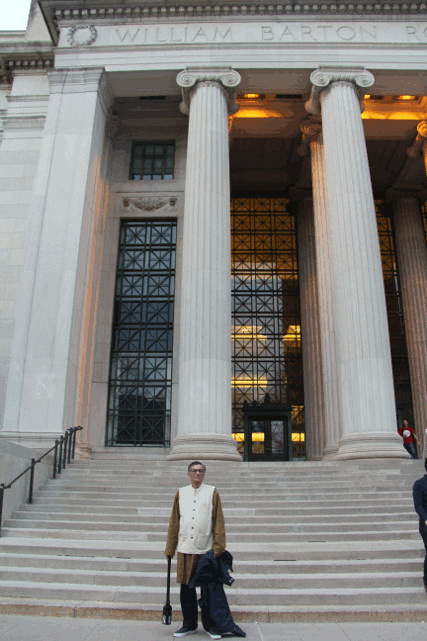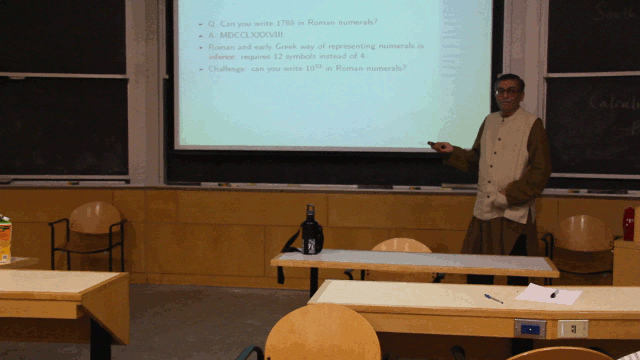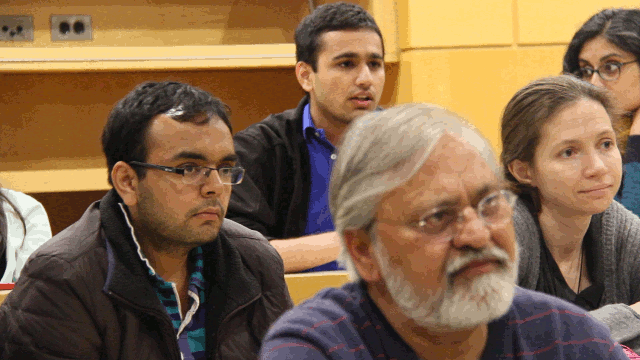## Archive for May, 2015

### MIT Talk: Calculus the real story

Tuesday, May 19th, 2015The abstract of my talk at MIT differs from my presentation, posted at http://ckraju.net/papers/presentations/MIT.pdf.

The point I made in the talk was that MIT teaches calculus and trigonometry wrongly. This is just because Europeans failed to understand the mathematics they imported from India. For example, the very words “trigonometry”, “zero”, “surd”, “sine”, indicate European conceptual mistakes and failure to understand imported Indian mathematics. We should therefore not blindly copy the MIT MOOC courses, but teach calculus differently, contrary to the recommendation made by Sam Pitroda.A video of the question and answer session would hopefully soon be online.### Raju’s paradox: all formal mathematicians are fools

Tuesday, May 12th, 2015

For almost two decades now, I have been pointing out that formal mathematics (and much Western philosophy) is based on the false belief that proof based on two valued logic (”deduction”) is “superior” to empirical proof (”induction”). This belief is NOT universal (e.g. Indian mathematicians used empirical proofs, e.g. Buddhists used a different logic), it is NOT empirically certain (e.g. quantum logic), it is anchored in Crusading myth (e.g. there was no “Euclid”, and the book Elements begins with an EMPIRICAL proof, and ends with an EMPIRICAL proof of the “Pythagorean” “theorem”). Formalism, the prevailing philosophy of mathematics, arose from an attempt to “save” that rotten myth that Westerners did something “superior” in math. This math has religious roots in bad Crusading theology (Aquinas’ bowdlerization of al Ghazali that logic binds God). All this mythology and theology has NOTHING to do with any practical application of mathematics, mostly done on computers today, and can be safely eliminated (e.g. using zeroism) without diminishing the practical value of math by an iota. Doing so makes math easy, and actually improves science. That is, it leads to a truly superior math.

At a recent conference in Vizag, I even tried to initiate a public discussion on this, which mathematicians have avoided so far. However, a discussion did take place, and the draft minutes are posted at http://ckraju.net/issa/conversation-draft-minutes.html.

In a more recent conversation with an award-winning formal mathematician, I was asked to give a concrete example of how formal mathematics can lead to wrong conclusions. I have already given examples such as the Banach-Tarski paradox, but they involve technicalities. So, here is a simple example.

Theorem: All formal mathematicians are fools.

Proof. Step 1. If Schrodinger’s cat is both alive and dead then all mathematicians are fools. (Instance of the tautology hence theorem of 2-valued logic that “A and not-A implies B”, where A and B are any propositions whatsoever.)

Step 2. It is an empirical fact that Schrodinger’s cat is both alive and dead. (More precisely, the cat is a macrophysical metaphor for an electron in the two-slit diffraction experiment: the electron both passes through a given slit 1 (A), and does not pass through it, i.e., passes through slit 2 (not-A). For more details see The Eleven Pictures of Time.)

Step 3. Therefore, all formal mathematicians are fools. (By modus ponens.)

QED